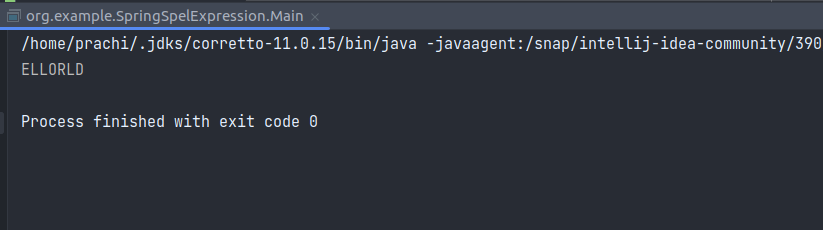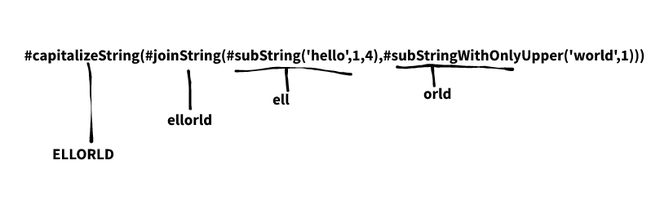Open in App
Not now

# Parse Nested User-Defined Functions using Spring Expression Language (SpEL)

• Difficulty Level : Expert
• Last Updated : 20 Dec, 2022

If we want to get the result of the function in java,

`convert(a,process(covertToInt(b),convertToInt(c)))`

what is the tool or solution we can refer to? The answer is here.

SpEL, or Spring Expression Language, is a potent expression language that allows for the runtime querying and manipulation of an object network. Although SpEL is a foundation for expression evaluation within the Spring portfolio, it is unrelated to Spring and can be utilized separately. The usage of SpEL to evaluate variables and parse expressions is covered in a plethora of educational articles and blog postings. However, a unique application of it is explained here. The ability to execute user-defined functions is one of the many applications that SpEL offers. The method registerFunction of the StandardEvaluationContext can be used to register a pre-written function.

Syntax:

`public void registerFunction(String name, Method m)`

### Implementation

Two files will be used to demonstrate the use case.

1. StringUtils.java – where all the functions are written.
2. Main.java – main class of the application.

## Java

 `import` `java.util.Locale; ` ` `  `public` `class` `StringUtils { ` ` `  `    ``public` `static` `String reverseString(String input) ` `    ``{ ` `        ``StringBuilder backwards = ``new` `StringBuilder(); ` `        ``for` `(``int` `i = ``0``; i < input.length(); i++) { ` `            ``backwards.append( ` `                ``input.charAt(input.length() - ``1` `- i)); ` `        ``} ` `        ``return` `backwards.toString(); ` `    ``} ` `    ``public` `static` `String capitalizeString(String input) ` `    ``{ ` `        ``return` `input.toUpperCase(Locale.ROOT); ` `    ``} ` ` `  `    ``public` `static` `String subString(String input, ``int` `start, ` `                                   ``int` `end) ` `    ``{ ` `        ``return` `input.substring(start, end); ` `    ``} ` ` `  `    ``public` `static` `String subString(String input, ``int` `start) ` `    ``{ ` `        ``return` `input.substring(start); ` `    ``} ` ` `  `    ``public` `static` `String joinString(String input1, ` `                                    ``String input2) ` `    ``{ ` `        ``return` `input1.concat(input2); ` `    ``} ` `}`

Here, The subString function is being overloaded and other functions are created normally.

## Java

 `package` `org.example.SpringSpelExpression; ` ` `  `import` `org.springframework.expression.*; ` `import` `org.springframework.expression.spel.standard.SpelExpressionParser; ` `import` `org.springframework.expression.spel.support.StandardEvaluationContext; ` ` `  `public` `class` `Main { ` `    ``public` `static` `void` `main(String[] args) ` `        ``throws` `NoSuchMethodException ` `    ``{ ` `        ``ExpressionParser parser ` `            ``= ``new` `SpelExpressionParser(); ` `        ``StandardEvaluationContext context ` `            ``= ``new` `StandardEvaluationContext(); ` ` `  `        ``context.registerFunction( ` `            ``"reverseString"``, ` `            ``StringUtils.``class``.getDeclaredMethod( ` `                ``"reverseString"``, String.``class``)); ` ` `  `        ``context.registerFunction( ` `            ``"capitalizeString"``, ` `            ``StringUtils.``class``.getDeclaredMethod( ` `                ``"capitalizeString"``, String.``class``)); ` ` `  `        ``context.registerFunction( ` `            ``"subString"``, ` `            ``StringUtils.``class``.getDeclaredMethod( ` `                ``"subString"``, String.``class``, ``int``.``class``, ` `                ``int``.``class``)); ` ` `  `        ``context.registerFunction( ` `            ``"subStringWithOnlyUpper"``, ` `            ``StringUtils.``class``.getDeclaredMethod( ` `                ``"subString"``, String.``class``, ``int``.``class``)); ` ` `  `        ``context.registerFunction( ` `            ``"joinString"``, ` `            ``StringUtils.``class``.getDeclaredMethod( ` `                ``"joinString"``, String.``class``, String.``class``)); ` ` `  `        ``String helloWorldReversed ` `            ``= parser ` `                  ``.parseExpression( ` `                      ``"#capitalizeString(#joinString(#subString('hello',1,4),#subStringWithOnlyUpper('world',1)))"``) ` `                  ``.getValue(context, String.``class``); ` ` `  `        ``System.out.println(helloWorldReversed); ` `    ``} ` `}`

All the functions are registered in the context using,

• Method name
• Method declared class
• Datatype of parameters of the method

As a result of the information provided above, method overloading is possible because the context may differentiate between methods not only by their names but also by the types of their parameters. In case of method overloading, the name in registerFunction must be distinct as shown above. The parseExpression method gives you the final result.

Output:### Flow of the Executionexecution flow

### Conclusion

When you do not want to pass the functions as parameters and break the execution manually, this dynamic solution can save your day.

My Personal Notes arrow_drop_up
Related Articles